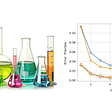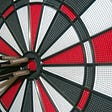# Building a Classifier from Scratch

`# import librariesimport numpy as npimport matplotlib.pyplot as plt%matplotlib inlineimport pandas as pdimport seaborn as snsfrom sklearn.model_selection import train_test_split, cross_val_score, GridSearchCVfrom sklearn.preprocessing import OneHotEncoder, LabelEncoder, StandardScalerfrom imblearn.over_sampling import SMOTEfrom sklearn.metrics import accuracy_score, f1_score, precision_score, recall_score, roc_curve, aucfrom sklearn import treefrom sklearn.tree import DecisionTreeClassifierfrom sklearn.neighbors import KNeighborsClassifierfrom sklearn.ensemble import RandomForestClassifier`
`# plot boxplotsplt.figure(figsize=(30, 10))df.boxplot()plt.tight_layout()plt.title('Boxplots of Numeric Columns')plt.xlabel('Numeric Features')plt.show()`
`plt.figure(figsize=(15, 5))sns.countplot(df['state'], color='red')plt.tight_layout()plt.title('State Counts')plt.show()`
`state_crosstab = pd.crosstab(df['state'], df['churn'], normalize='index')state_crosstab`
`# sort the plot by churn rate to get a better sense of which states are associated with higher churnsorted_state_churn = state_crosstabsorted_state_churn = sorted_state_churn.reset_index()sorted_state_churn.columns = ['state', 'churn_rate']# sort values based on churn ratesorted_state_churn.sort_values('churn_rate', inplace=True)# plot and highlight states with churn rates higher than 20%plt.figure(figsize=(15, 5))ax = sns.barplot(sorted_state_churn['state'], sorted_state_churn['churn_rate'], color='red')plt.title("Sorted Churn Rate by State")for bar in ax.patches:    if bar.get_height() >= 0.20:        bar.set_color('red')    else:        bar.set_color('grey')plt.show()`
`# International Planint_plan_crosstab = pd.crosstab(df['international plan'], df['churn'])normalized_int_plan_crosstab = pd.crosstab(df['international plan'], df['churn'], normalize='index')print('International Plan Counts:')display(int_plan_crosstab)print('--------------------------------')print('International Plan %:')display(normalized_int_plan_crosstab)`
`# plot boxplotsfig, axes = plt.subplots(nrows=8, ncols=2, figsize=(15, 15))for ax, feat in zip(axes.flatten(), num_cols):    sns.boxplot(x=feat, y=df['churn'].astype('category'), data=df, ax=ax)    ax.set_title(f'{feat} vs. churn')plt.tight_layout()plt.show()`
`# plot a heatmap of correlationscorr = df.corr()plt.figure(figsize=(15,10))sns.heatmap(corr, cmap='RdBu_r', annot=False, vmax=1, vmin=-1)plt.show()`
`# creation of new variablesdf['all_non_intl_calls'] = df['total day calls'] + df['total eve calls'] + df['total night calls']df['all_calls'] = df['all_non_intl_calls'] + df['total intl calls']df['all_non_intl_mins'] = df['total day minutes'] + df['total eve minutes'] + df['total night minutes']df['all_mins'] = df['all_non_intl_mins'] + df['total intl minutes']df['avg_non_intl_call_time'] = df['all_non_intl_mins'] / df['all_non_intl_calls']df['avg_call_time'] = df['all_mins'] / df['all_calls']`
`predictors = ['state', 'account length', 'international plan', 'voice mail plan',              'number vmail messages', 'total day minutes', 'total eve minutes',               'total night minutes', 'total intl minutes', 'customer service calls',               'all_non_intl_calls', 'all_calls', 'all_non_intl_mins', 'all_mins',               'avg_non_intl_call_time', 'avg_call_time']`
`X = clean_df[predictors]y = clean_df['churn']final_clean_df = pd.concat([X, y], axis=1)# create train and test sets, stratify to keep proportionsX_train, X_test, y_train, y_test = train_test_split(X, y, random_state=SEED, stratify=y)`
`# encode columns# stateohe = OneHotEncoder(categories='auto', handle_unknown='ignore', sparse=False)X_train_state_encoded = ohe.fit_transform(X_train[['state']])X_train_state_encoded = pd.DataFrame(X_train_state_encoded, columns=ohe.categories_, index=X_train.index)X_test_state_encoded = ohe.transform(X_test[['state']])X_test_state_encoded = pd.DataFrame(X_test_state_encoded, columns=ohe.categories_, index=X_test.index)# drop initial state column from both test and train data, and concat with remaining featuresX_train = X_train.drop('state', axis=1)X_test = X_test.drop('state', axis=1)X_train_ohe = pd.concat([X_train, X_train_state_encoded], axis=1)X_test_ohe = pd.concat([X_test, X_test_state_encoded], axis=1)# label encode voice mail plan and international planle = LabelEncoder()# encode international planX_train_ohe['intl_plan_encoded'] = le.fit_transform(X_train_ohe['international plan'])X_test_ohe['intl_plan_encoded'] = le.transform(X_test_ohe['international plan'])# encode voicemail planX_train_ohe['vm_plan_encoded'] = le.fit_transform(X_train_ohe['voice mail plan'])X_test_ohe['vm_plan_encoded'] = le.transform(X_test_ohe['voice mail plan'])# drop original columnsX_train_encoded = X_train_ohe.drop(['international plan', 'voice mail plan'], axis=1)X_test_encoded = X_test_ohe.drop(['international plan', 'voice mail plan'], axis=1)X_train_final = X_train_encoded.copy()X_test_final = X_test_encoded.copy()`
`# print initial target class weightsprint('Initial Class Weights of Target:')print(y_train.value_counts())print(y_train.value_counts(normalize=True))`
`# create synthetic training data using SMOTE to address imbalanceX_train_resampled, y_train_resampled = SMOTE(random_state=SEED).fit_resample(X_train_final, y_train)X_train_resampled = pd.DataFrame(X_train_resampled, columns=X_train_final.columns)# print new class weightsprint('Balanced Class Weights of Target:')print(pd.Series(y_train_resampled).value_counts())print(pd.Series(y_train_resampled).value_counts(normalize=True))`
`def print_model_scores(X_train, X_test, y_train, y_test, model, model_name):    """    Function to return accuracy, recall, precision, f1, roc_auc, and neg_log_loss from given X_train, X_test, y_train, y_test, and fit model.    """    # create predictions using model    y_test_preds = model.predict(X_test)    y_train_preds = model.predict(X_train)        # accuracy scores    train_accuracy = accuracy_score(y_train, y_train_preds)    test_accuracy = accuracy_score(y_test, y_test_preds)        # precision score    train_precision = precision_score(y_train, y_train_preds)    test_precision = precision_score(y_test, y_test_preds)        # recall score    train_recall = recall_score(y_train, y_train_preds)    test_recall = recall_score(y_test, y_test_preds)        # f1 score    train_f1 = f1_score(y_train, y_train_preds)    test_f1 = f1_score(y_test, y_test_preds)        # roc_auc    false_positive_rate, true_positive_rate, thresholds = roc_curve(y_test, y_test_preds)    test_roc_auc = auc(false_positive_rate, true_positive_rate)    fpr, tpr, thresh = roc_curve(y_train, y_train_preds)    train_roc_auc = auc(fpr, tpr)        print('Accuracy:')    print(f'Training Set: {train_accuracy}')    print(f'Test Set: {test_accuracy}')    print('---------------------------------')    print('Precision:')    print(f'Training Set: {train_precision}')    print(f'Test Set: {test_precision}')    print('---------------------------------')    print('Recall:')    print(f'Training Set: {train_recall}')    print(f'Test Set: {test_recall}')    print('---------------------------------')    print('F1 Score:')    print(f'Training Set: {train_f1}')    print(f'Test Set: {test_f1}')    print('---------------------------------')    print(f'ROC AUC:')    print(f'Training Set: {train_roc_auc}')    print(f'Test Set: {test_roc_auc}')        test_results = pd.DataFrame([[f'Test-{model_name}', test_accuracy, test_precision, test_recall, test_f1, test_roc_auc]],                                columns=['Model', 'Accuracy', 'Precision', 'Recall', 'F1 Score', 'AUC'])        train_results = pd.DataFrame([[f'Training-{model_name}', train_accuracy, train_precision, train_recall, train_f1, train_roc_auc]],                                columns=['Model', 'Accuracy', 'Precision', 'Recall', 'F1 Score', 'AUC'])        # concat results    results = pd.concat([test_results, train_results], axis=0)        return results`
`# run a SVC and see if we can improve scoringfrom xgboost import XGBClassifier# create baseline classifierbaseline_xgb = XGBClassifier(random_state=SEED)# fitbaseline_xgb.fit(X_train_resampled, y_train_resampled)# store scoresbaseline_xgb_results = print_model_scores(X_train_resampled,                                          X_test_final,                                          y_train_resampled,                                          y_test,                                          baseline_xgb,                                          'baseline_xgb')`
`xgb_param_grid = {    'learning_rate': [0.1, 0.2],    'max_depth': [1, 2, 3],    'subsample': [0.5, 0.7],    'min_child_weight': [2, 3]}# create gridsearchxgb_grid_search = GridSearchCV(baseline_xgb,                               xgb_param_grid,                               cv=3,                               return_train_score=True,                               scoring='f1')# fit modelxgb_grid_search.fit(X_train_resampled, y_train_resampled)`
`# Mean training scorexgb_gs_training_score = np.mean(xgb_grid_search.cv_results_['mean_train_score'])# Mean test scorexgb_gs_testing_score = xgb_grid_search.score(X_test_final, y_test)print(f'Mean Training Score: {xgb_gs_training_score}')print(f'Mean Testing Score: {xgb_gs_testing_score}')print("Best Parameter Combination Found During Grid Search:")xgb_grid_search.best_params_`
`# train model with these parametersbest_xgb = XGBClassifier(random_state=SEED,                         learning_rate=0.2,                         max_depth=3,                         subsample=0.5,                         min_child_weight=2)# fit to training databest_xgb.fit(X_train_resampled, y_train_resampled)# store resultsbest_xgb_results = print_model_scores(X_train_resampled,                                       X_test_final,                                      y_train_resampled,                                      y_test,                                      best_xgb,                                      'best_xgb')`
`all_predictions = final_model.predict(transformed_data)all_probs = final_model.predict_proba(transformed_data)`

--

--

--

## More from Kai Graham

Love podcasts or audiobooks? Learn on the go with our new app.

## Data Science is beyond Regression and Classification## Starting guide to artificial intelligence part 2## Using Neural Networks to Boost Student Learning in Chemistry## Top 4 Skills A Data Analyst Must Have## Testing if two graphs are isomorphic## A COVID Critic’s Guide: Will Flattening the Curve Really Be Enough?## Hyperparameter tuning guide## Music Genre Prediction using ML and Optuna## Managing Machine Learning Lifecycles with MLflow## Expected value as evaluation metric in Machine Learning